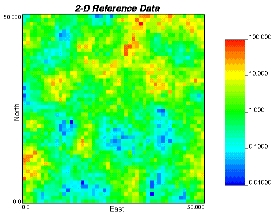GSLIB Help Page: PIXELPLTDescription:
• For plotting 2-D slices through gridded 3-D data sets, e.g., as generated by kriging or simulation algorithms, one can use gray or color scale maps.Parameters:
• datafl: The data file containing the 2-D/3-D grids with x cycling fastest, then y then z then per simulation.
• icol: column number in the input file to consider. This is typically one (1).
• tmin and tmax: all values strictly less than tmin and strictly greater than tmax are ignored.
• outfl: file for PostScript output.
• ireal: the grid or realization number to consider.
• nx, xmn, and xsiz: the number of nodes in the x direction, the origin, and the grid node separation.
• ny, ymn, and ysiz: the number of nodes in the y direction, the origin, and the grid node separation.
• nz, zmn, and zsiz: the number of nodes in the z direction, the origin, and the grid node separation.
• slice: the type of slice through the 3-D grid to consider (1=horizontal XY slice, 2=vertical XZ slice, 3=vertical YZ slice). The axes increase to the right and to the top.
• sliceno: the slice number to consider (if slice=1 then sliceno must be between 1 to nz, if slice=2 then sliceno must be between 1 to ny, if slice=3 then sliceno must be between 1 to nx.
• title: a 40-character title for the top of the plot.
• xlab: a 40-character label that will appear centered below the plot.
• ylab: a 40-character label that will appear vertically centered to the left of the plot.
• ilog: =0 then an arithmetic scaling of the gray/color scale is used, =1 then a logarithmic scaling (base 10) is used.
• igray: =0 then a gray scale is used, =1 then a color scale is used.
• icont: =0 for a continuous variable, =1 for a categorical variable.
• gmin, gmax, and ginc: minimum, maximum, and labeling increment for a continuous variable. These do not have to be compatible with tmin and tmax Values that are less than gmin or greater than gmax will appear just beyond either end of the gray/color spectrum.
• ncat: number of categories for each category:
• code, colorcode, and name: the integer code of the category, the color code to plot the category, and a name to put beside the color on the legend. The color codes have been chosen arbitrarily as follows: 1=red, 2=orange, 3=yellow, 4=light green, 5=green, 6=light blue, 7=dark blue, 8=violet, 9=white, 10=black, 11=purple, 12=brown, 13=pink, 14=intermediate green, and 15=gray.